# JP5367552B2 - Image processing apparatus and image processing program - Google Patents

## Info

Publication number
JP5367552B2
JP5367552B2 JP2009284317A JP2009284317A JP5367552B2 JP 5367552 B2 JP5367552 B2 JP 5367552B2 JP 2009284317 A JP2009284317 A JP 2009284317A JP 2009284317 A JP2009284317 A JP 2009284317A JP 5367552 B2 JP5367552 B2 JP 5367552B2
Authority
JP
Japan
Prior art keywords
bits
rgb
value
format
image processing
Prior art date
Legal status (The legal status is an assumption and is not a legal conclusion. Google has not performed a legal analysis and makes no representation as to the accuracy of the status listed.)
Active
Application number
JP2009284317A
Other languages
Japanese (ja)
Other versions
JP2011128714A (en
Inventor

Original Assignee

Priority date (The priority date is an assumption and is not a legal conclusion. Google has not performed a legal analysis and makes no representation as to the accuracy of the date listed.)
Filing date
Publication date
Application filed by 株式会社東芝 filed Critical 株式会社東芝
Priority to JP2009284317A priority Critical patent/JP5367552B2/en
Publication of JP2011128714A publication Critical patent/JP2011128714A/en
Application granted granted Critical
Publication of JP5367552B2 publication Critical patent/JP5367552B2/en
Application status is Active legal-status Critical
Anticipated expiration legal-status Critical

• 238000000034 methods Methods 0 abstract 1
• 238000003860 storage Methods 0 abstract 1

## Images

•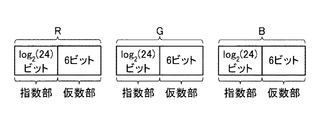•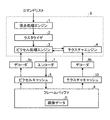•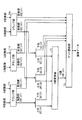••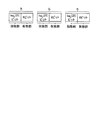•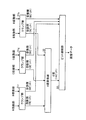•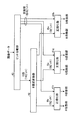•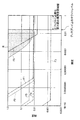## Classifications

• HELECTRICITY
• H04ELECTRIC COMMUNICATION TECHNIQUE
• H04NPICTORIAL COMMUNICATION, e.g. TELEVISION
• H04N9/00Details of colour television systems
• H04N9/64Circuits for processing colour signals
• H04N9/68Circuits for processing colour signals for controlling the amplitude of colour signals, e.g. automatic chroma control circuits
• HELECTRICITY
• H03BASIC ELECTRONIC CIRCUITRY
• H03MCODING; DECODING; CODE CONVERSION IN GENERAL
• H03M7/00Conversion of a code where information is represented by a given sequence or number of digits to a code where the same, similar or subset of information is represented by a different sequence or number of digits
• H03M7/14Conversion to or from non-weighted codes
• H03M7/24Conversion to or from floating-point codes

## Abstract

Image processing apparatus of the embodiments includes a storage which stores data on any of color and brightness of a pixel represented in a first floating-point format including a significand represented in an integer-bit length and an exponent represented in a non-integer-bit length; and an image processor which processes the data.

## Description

The present invention relates to an image processing equipment Contact and image processing program, in particular, it is suitably applied to a format for representing a color or brightness of pixels in the floating point format.

In order to generate high-quality computer graphics images, the importance of HDR (High Dynamic-range Rendering) technology is increasing. In this HDR, it is necessary to process with a wide dynamic range exceeding the range of 0 to 1.0 with respect to the color of each pixel.

Therefore, a format (R16G16B16A16_FLOAT) that expresses the brightness of each RGB component of each pixel in the frame buffer in a 16-bit floating point format (exponent part 5 bits, mantissa part 11 bits) is widely used. However, in this method, 3 × 16 bits are required to express the brightness of each RGB component. For this reason, if an alpha component representing transparency is included, only 64 bits are consumed per pixel, and the memory capacity and memory bandwidth increase.

In order to halve the memory capacity and the memory bandwidth, several methods of expressing with 32 bits per pixel have been proposed. For example, Microsoft DirectX10 defines floating point base formats such as R9G9B9E5_SHAREDEXP and R11G11B10_FLOAT. Further, Xbox 360 of a commercial game machine uses a format of R10G10B10A2_FLOAT.

Further, for example, Patent Document 1 discloses that an image is decomposed into a plurality of image blocks, pixel color information and luminance information in the image block are separated from a high dynamic range value of each pixel, and pixel color information in the image block A method of compressing pixel luminance information in an image block independently of each other is disclosed.

JP 2009-524315 A

However, in R9G9B9E5_SHAREDEXP, there is a problem that the accuracy is extremely lowered when the color is saturated because the exponent part is common to the RGB components.

In addition, R11G11B10_FLOAT has a problem that the mantissa part of the B channel is as small as 5 bits and a Mach band may be perceived.

In addition, in R10G10B10A2_FLOAT, although the encoding and decoding circuit scale is small, only 3 bits are allocated to the exponent part, so that the dynamic range is insufficient and the accuracy is insufficient, and the Mach band may be perceived. There was a problem.

The method disclosed in Patent Document 1 relates to compression of image data expressed in various formats, and does not relate to a format expression for reducing memory capacity and memory bandwidth.

An object of the present invention, while reducing the number of bits allocated per pixel, offers a wide while securing the dynamic range, image processing program and our image processing equipment capable of Mach bands from being perceived It is to be.

According to one aspect of the present invention, the color or brightness of a pixel is expressed in a floating-point format of a first format including a mantissa part represented by an integer bit length and an exponent part represented by a non-integer bit length. Provided is an image processing apparatus comprising a storage unit for storing data and an image processing unit for processing the data.

According to one aspect of the present invention, image data represented in a floating-point format including a mantissa part in which the color or brightness of a pixel is represented by an integer bit length and an exponent part represented by a non-integer bit length is obtained. An image processing program is provided that causes a computer to execute a step of storing and a step of processing the image data.

According to the present invention, it is possible to secure a wide dynamic range and reduce the perception of a Mach band while reducing the number of bits allocated per pixel.

FIG. 1 is a diagram showing an example of a format for expressing the brightness of each element of RGB according to the first embodiment of the present invention in a floating-point format. FIG. 2 is a block diagram showing a schematic configuration of an image processing apparatus according to the second embodiment of the present invention. FIG. 3 is a diagram illustrating an example of the encoder 7 of FIG. 2 corresponding to the format of FIG. 4 is a diagram illustrating an example of the decoders 6 and 8 of FIG. 2 corresponding to the format of FIG. FIG. 5 is a view showing an example of a format for expressing the brightness of each color of RGB according to the third embodiment of the present invention in a floating-point format. 6 is a diagram illustrating an example of the encoder 7 in FIG. 2 corresponding to the format in FIG. 7 is a diagram illustrating an example of the decoders 6 and 8 of FIG. 2 corresponding to the format of FIG. FIG. 8 is a diagram showing the relationship between brightness and accuracy expressed in the format according to the embodiment of the present invention in comparison with the conventional format.

Hereinafter, a format and an image processing apparatus used for expressing image data according to an embodiment of the present invention will be described with reference to the drawings. In the following description, a case where the pixel color is expressed by three RGB elements will be described as an example. However, the present invention is not limited to the case where the pixel color is expressed by three RGB elements. For example, the present invention may be applied to a case where the pixel color is expressed by three YUV elements.

(First embodiment)
FIG. 1 is a diagram showing an example of a format for expressing the brightness of each color of RGB according to the first embodiment of the present invention in a floating-point format.
In FIG. 1, in this format, the brightness of each color of RGB is expressed in a floating-point format with a mantissa part 6 bits and an exponent part log 2 (24) bits (about 4.585 bits).
Here, in the exponent part of log 2 (24) bits, a range of m * 2 n to m * 2 n + 23 can be expressed. However, n is an arbitrary integer, and m is a value of the mantissa part.
The exponent parts of each RGB color expressed in the format of FIG. 1 can be encoded together to 14 bits.

That is, since the relationship 3 * log 2 (24) = log 2 (3 3 * 2 9 ) <log 2 (2 14 ) is satisfied, the information amount of the exponent part for the three colors of RGB is suppressed to 14 bits or less. Can do.

As a result, even when 6 bits are assigned to the mantissa part of each color of RGB, the number of bits assigned to the exponent part of each color of RGB is reduced while keeping the number of bits assigned to one pixel to 32 bits or less. Can be suppressed. Therefore, it is possible to ensure a wide dynamic range and prevent the Mach band from being perceived while suppressing an increase in memory capacity and memory bandwidth.

When the exponent part is 0, the mantissa part may be treated as a denormalized number. In this case, the minimum resolution can be enlarged 2 n-5, and the dynamic range into 2 n + 23/2 n- 5 = 2 28.

(Second Embodiment)
FIG. 2 is a block diagram showing a schematic configuration of an image processing apparatus according to the second embodiment of the present invention.
In FIG. 2, the image processing apparatus includes an image processing unit 9, decoders 6a and 6b, an encoder 7, a pixel cache 5, a texture cache 10, and a frame buffer 8. The image processing unit 9 can process the image data D. The frame buffer 8 can store image data D processed by the image processing unit 9. The image data D can be expressed in a raster format using the format of FIG. The decoders 6 a and 6 b can convert the image data D expressed in the format of FIG. 1 into a format handled by the image processing unit 9. The encoder 7 can convert the image data D expressed in the format handled by the image processing unit 9 into the format shown in FIG. The pixel cache 5 can hold pixel data to which attributes are assigned. The pixel cache 5 can hold pixel data, for example, for each entry (rectangle). The texture cache 10 can temporarily hold the pixel attributes read from the frame buffer 8. Examples of pixel attributes include colors, patterns, and textures.

Here, the image processing unit 9 is provided with a vertex processing engine 1, a rasterizer 2, a pixel processing engine 3, and a texture engine 4. The vertex processing engine 1 can perform coordinate conversion of a vertex of a triangle representing a graphic. In addition, as coordinate conversion of a vertex, the coordinate conversion accompanying rotation, reduction, or expansion of the figure comprised by a vertex can be mentioned, for example. Further, the vertex processing engine 1 may convert the drawing command and drawing data (start point, end point, control point, etc.) in the command list into a plurality of triangles constituting the stroke. The drawing data of the command list can be expressed in a format other than the raster format such as a vector format. The rasterizer 2 can convert a triangle composed of vertices into a collection of pixels. The texture engine 4 can hold pixel attributes or perform processing related to pixel attributes. For example, the texture engine 4 can calculate a memory address from the texture coordinates or operate as a texture filter. Examples of pixel attributes include colors, patterns, and textures. The pixel processing engine 3 can generate an attribute to be given to a pixel by an arbitrary calculation process using the attribute acquired by the texture engine 4 as an input.

Then, in the vertex processing engine 1, the drawing command and drawing data in the command list are converted into a plurality of triangles constituting the stroke, and after the coordinate conversion of the vertices constituting the triangle is performed, it is input to the rasterizer 2. . In the rasterizer 2, the triangle formed by the vertices is converted into a collection of pixels and input to the pixel processing engine 3.

On the other hand, the image data D stored in the frame buffer 8 is output to the decoder 6b via the texture cache 10, converted from the format of FIG. 1 into a format handled by the image processing unit 9, and then sent to the texture engine 4. . Then, after processing related to pixel attributes is performed in the texture engine 4, it is output to the pixel processing engine 3. The image data D stored in the frame buffer 8 is output to the decoder 6a via the pixel cache 5 and converted from the format shown in FIG. 1 into a format handled by the image processing unit 9, and then sent to the pixel processing engine 3. It is done.

Then, in the pixel processing engine 3, the attribute finally given to the pixel is calculated and sent to the encoder 7. Then, in the encoder 7, the image data D is updated by overwriting the image data D stored in the pixel cache 5 after being converted from the format handled by the image processing unit 9 to the format shown in FIG. 1. Is done. The image data D on the pixel cache 5 updated by the pixel processing engine 3 is stored in the frame buffer 8.

FIG. 3 is a diagram showing an example of the encoder 7 of FIG. 2 corresponding to the format of FIG.
3, the encoder 7 of FIG. 2 is provided with clamp portions 11r, 11g, 11b, an E value converter 12, and a bit connecting portion 13. The clamp units 11r, 11g, and 11b can clamp the values of the RGB elements handled by the image processing unit 9 (hereinafter referred to as RGB values) in a range that can be expressed in the format of FIG. In addition, you may make it the clamp parts 11r, 11g, and 11b perform the process which converts a mantissa part into a denormalized number as needed. The E value converter 12 can convert the upper 2 bits of the exponent part of each RGB value into a 5-bit compressed exponent code (referred to as an E value). The E value converter 12 may be configured by a group of arithmetic units or a table. The bit concatenation unit 13 concatenates the 5-bit E value obtained by the E-value converter 12, the lower 3 bits of the exponent part of each RGB value, and the 6 bits of the mantissa part of each RGB value. , 32-bit values can be generated. Each RGB value input to the encoder 7 is a floating point number.

When the RGB values are input to the clamp units 11r, 11g, and 11b, the RGB values are clamped to a range that can be expressed in the format of FIG. And the lower 3 bits of the exponent part of each RGB value and the 6 bits of the mantissa part of each RGB value are output to the bit concatenation unit 13. Then, the E value converter 12 converts the upper 2 bits of the exponent part of each RGB value into a 5-bit E value and outputs it to the bit concatenation unit 13. The bit concatenation unit 13 concatenates the 5-bit E value obtained by the E-value converter 12, the lower 3 bits of the exponent part of each RGB value, and the 6 bits of the mantissa part of each RGB value. Image data D expressed in the format of FIG. 1 is generated.

Specifically, in the clamp units 11r, 11g, and 11b, the exponents n to n + 23 of the RGB values are represented by binary codes of 0 to 23, and are represented by 5 bits for each RGB element. Here, assuming that the upper 2 bits of the exponent part of each value of RGB are Re, Ge, and Be, the E value converter 12 performs, for example, a calculation of E = Re * 3 2 + Ge * 3 + Be to obtain 5 bits. E value can be obtained. The relationship between Re, Ge, and Be can be arbitrarily changed. In addition, since the range which Re, Ge, and Be can take is 0-2, the range of E becomes 0-26. For this reason, E can be expressed by 5 bits, and when the lower 3 bits of the exponent part of each value of RGB are combined, it becomes 14 bits as a whole.

Here, by expressing the exponent part of the brightness of each color of RGB with log 2 (24) bits, the upper 2 bits and the lower 3 bits of the exponent part can be handled separately. For this reason, the E value converter 12 can be 6-bit input and 5-bit output, and the configuration of the E value converter 12 can be simplified.

FIG. 4 is a diagram showing an example of the decoders 6 and 8 of FIG. 2 corresponding to the format of FIG.
In FIG. 4, the decoders 6 and 8 of FIG. 2 are provided with normalization units 21r, 21g, and 21b, an E value inverse converter 22 and a bit separation unit 23. The bit separation unit 23 converts the 32-bit value expressed in the format of FIG. 1 into a 5-bit E value, lower 3 bits of the exponent part of each RGB value, and 6 bits of the mantissa part of each RGB value. Can be separated. The E value inverse converter 22 can inversely convert the 5-bit E value into the upper 2 bits of the exponent part of each RGB value. Note that the E-value inverse converter 22 may be composed of a computing unit group or a table. Normalizers 21r, 21g, and 21b are image processing units that convert the upper 2 bits of the exponent part of each RGB value, the lower 3 bits of the exponent part of each RGB value, and the 6 bits of the mantissa part of each RGB value. 9 can be converted into RGB values.

When the image data D expressed in the format of FIG. 1 is input to the bit separation unit 23, the 5-bit E value, the lower 3 bits of the exponent part of each RGB value, and the mantissa of each RGB value And the 5-bit E value is output to the E-value inverse converter 22, and the lower 3 bits of the exponent part of each RGB value and the 6 bits of the mantissa part of each RGB value are normalized. Are output to the conversion units 21r, 21g, and 21b, respectively.

Then, the E value inverse converter 22 inversely converts the 5-bit E value into the upper 2 bits of the exponent part of each RGB value, and outputs it to the normalization units 21r, 21g, and 21b. Then, in the normalization units 21r, 21g, and 21b, the upper 2 bits of the exponent part of each RGB value, the lower 3 bits of the exponent part of each RGB value, and the 6 bits of the mantissa part of each RGB value Then, normalization is performed in accordance with the floating-point format and output to the image processing unit 9.

(Third embodiment)
FIG. 5 is a diagram showing an example of a format that expresses the brightness of each color of RGB according to the third embodiment of the present invention in a floating-point format.
In FIG. 5, in this format, the brightness of each color of RGB is expressed in a floating-point format with a mantissa part 6 bits and an exponent part log 2 (25) bits (about 4.644 bits).
Here, in the exponent part of log 2 (25) bits, a range of m * 2 n to m * 2 n + 24 can be expressed. However, n is an arbitrary integer, and m is a value of the mantissa part.
The exponent part of each color of RGB expressed in the format of FIG. 5 can be collectively encoded to 14 bits.

That is, since the relationship 3 * log 2 (25) = log 2 (5 6 ) <log 2 (2 14 ) is satisfied, the information amount of the exponent part for the three colors of RGB can be suppressed to 14 bits or less.

As a result, even when 6 bits are assigned to the mantissa part of each color of RGB, the decrease in the number of bits assigned to the exponent part of each color of RGB is suppressed while keeping the number of bits assigned to each pixel to 32 bits or less. can do. Therefore, it is possible to ensure a wide dynamic range and prevent the Mach band from being perceived while suppressing an increase in memory capacity and memory bandwidth.

When the exponent part is 0, the mantissa part may be treated as a denormalized number. In this case, the minimum resolution can be enlarged 2 n-5, and the dynamic range into 2 n + 24/2 n- 5 = 2 29.

FIG. 6 is a diagram illustrating an example of the encoder 7 of FIG. 2 corresponding to the format of FIG.
6, the encoder 7 of FIG. 2 is provided with clamp portions 31r, 31g, 31b, an E value converter 32, and a bit connecting portion 33. The clamp units 31r, 31g, and 31b can clamp RGB values handled by the image processing unit 9 to a range that can be expressed in the format of FIG. The clamp units 31r, 31g, and 31b may perform a process of converting the mantissa part into a denormalized number as necessary. The E value converter 32 can convert 5 bits of the exponent part of each RGB value into a 14-bit E value. The E value converter 32 is equivalent to a radix converter that converts a 25-digit number into a binary number, and may be configured by a group of arithmetic units or a table. The bit concatenation unit 33 can generate a 32-bit value by concatenating the 14-bit E value obtained by the E-value converter 32 and the 6-bit mantissa of each RGB value.

When the RGB values are input to the clamp units 31r, 31g, and 31b, the RGB values are clamped to a range that can be expressed in the format shown in FIG. At the same time, 6 bits of the mantissa part of each RGB value are output to the bit concatenation unit 33. Then, the E value converter 32 converts the 5 bits of the exponent part of each RGB value into a 14-bit E value and outputs it to the bit concatenation unit 33. Then, the bit concatenation unit 33 concatenates the 14-bit E value obtained by the E value converter 32 and the 6 bits of the mantissa part of each RGB value, and the image data D expressed in the format of FIG. Generated.

Specifically, in the clamp units 31r, 31g, and 31b, the indices n to n + 24 of the RGB values are represented by binary codes of 0 to 24, and are represented by 5 bits for each RGB element. Here, assuming that 5 bits of the exponent part of each value of RGB are Re, Ge, and Be, the E value converter 32 performs, for example, the calculation of E = Re * 25 2 + Ge * 25 + Be to obtain 14 bits. An E value can be obtained. The relationship between Re, Ge, and Be can be arbitrarily changed. Since the range that Re, Ge, and Be can take is 0 to 24, the range of E is 0 to 15624. Therefore, E can be expressed by 14 bits.

Here, in the format of FIG. 5, the E value converter 32 has a 15-bit input and a 14-bit output, but the configuration of the E value converter 12 of FIG. 3 is larger than that in the case of using the format of FIG. The dynamic range can be expanded as compared with the format of FIG.

FIG. 7 is a diagram showing an example of the decoders 6 and 8 of FIG. 2 corresponding to the format of FIG.
In FIG. 7, the decoders 6 and 8 of FIG. 2 are provided with normalization units 41r, 41g, and 41b, an E value inverse converter 42, and a bit separation unit 43. The bit separation unit 43 can separate the 32-bit value expressed in the format of FIG. 5 into a 14-bit E value and a 6-bit mantissa part of each RGB value. The E value inverse converter 42 can inversely convert the 14-bit E value into 5 bits of RGB values. Note that the E-value inverse converter 42 may be composed of a computing unit group or a table. The normalization units 41r, 41g, and 41b can convert 5 bits of the exponent part of each RGB value and 6 bits of the mantissa part of each RGB value to each RGB value handled by the image processing unit 9. .

Then, when the image data D expressed in the format of FIG. 5 is input to the bit separation unit 43, it is separated into a 14-bit E value and a 6-bit mantissa part of each RGB value, and a 14-bit E value. Are output to the E-value inverse converter 42, and 6 bits of the mantissa part of each RGB value are output to the normalization units 41r, 41g, and 41b, respectively.

Then, in the E-value inverse converter 42, the 14-bit E value is inversely converted into 5 bits of the exponent part of each RGB value and output to the normalization units 41r, 41g, and 41b, respectively. Then, in the normalization units 41r, 41g, and 41b, normalization is performed according to the floating-point format from the 5 bits of the exponent part of each RGB value and the 6 bits of the mantissa part of each RGB value, and the image processing unit 9 is output.

FIG. 8 is a diagram showing the relationship between brightness and accuracy expressed in the format according to the embodiment of the present invention in comparison with the conventional format. F1 is the relationship between the brightness and accuracy expressed in the format of FIG. 1, F2 is the relationship between the brightness and accuracy expressed in the format of FIG. 5, the relationship between the brightness and accuracy expressed in R16G16B16A16_FLOAT, and F4 is The relationship between brightness and accuracy expressed in R11G11B10_FLOAT (Red, Green), F5 indicates the relationship between brightness and accuracy expressed in R11G11B10_FLOAT (Blue), and F6 indicates the relationship between brightness and accuracy expressed in R10G10B10A2_FLOAT.

In FIG. 8, R16G16B16A16_FLOAT can secure a wide dynamic range with high accuracy, but consumes a capacity of 64 bits per pixel. In R11G11B10_FLOAT, since the mantissa part of the B channel is as small as 5 bits, a part of the region R where the Mach band is perceived is partially applied. In R10G10B10A2_FLOAT, since only 3 bits are assigned to the exponent part, a part of the region R where the Mach band is perceived is applied.

On the other hand, in the formats of FIGS. 1 and 5, a wider dynamic range can be ensured compared to R10G10B10A2_FLOAT while avoiding the region R where the Mach band is perceived.

The vertex processing engine 1, the rasterizer 2, the pixel processing engine 3, the texture engine 4, the decoders 6a and 6b, and the encoder 7 cause the computer to execute a program in which instructions for performing processing performed in these blocks are described. Can be realized.

If this program is stored in a storage medium such as a CD-ROM, the vertex processing engine 1, rasterizer 2, pixel is installed by installing the storage medium in the computer of the image processing apparatus and installing the program in the computer. The processing performed by the processing engine 3, the texture engine 4, the decoders 6a and 6b, and the encoder 7 can be realized.

Further, when a computer executes a program in which instructions for performing processing performed by the vertex processing engine 1, the rasterizer 2, the pixel processing engine 3, the texture engine 4, the decoders 6a and 6b, and the encoder 7 are executed, a stand-alone computer is used. It may be executed, or may be distributed to a plurality of computers connected to the network.

1 vertex processing engine, 2 rasterizer, 3 pixel processing engine, 4 texture engine, 5 pixel cache, 6a, 6b decoder, 7 encoder, 8 frame buffer, 9 image processing unit, 10 texture cache, 11r, 11g, 11b, 31r, 31g, 31b Clamp unit, 12, 32 E value converter, 13, 33 bit concatenation unit, 21r, 21g, 21b, 41r, 41g, 41b Normalization unit, 22, 42 E value inverse converter, 23, 43 bit separation Part

## Claims (12)

1. A storage unit for storing data represented in a floating-point format of a first format including a mantissa part in which the color or brightness of a pixel is represented by an integer bit length and an exponent part represented by a non-integer bit length;
An image processing apparatus comprising: an image processing unit that processes the data.
2. A decoding unit for converting data expressed in the first format into data expressed in a floating-point format of a second format including a mantissa part expressed in integer bit length and an exponent part expressed in integer bit length When,
The image processing apparatus according to claim 1, further comprising an encoding unit that converts data expressed in the second format into data in the first format.
3. The image processing unit
A vertex processing unit that performs coordinate conversion of the vertex of a triangle that represents a figure;
A rasterizing unit for converting a triangle composed of the vertices into a collection of pixels;
The image processing apparatus according to claim 1, further comprising: a pixel processing unit that assigns an attribute to the pixel.
4. The pixel color is expressed by three elements of RGB, and the lightness of each RGB element includes a mantissa part designated by 6 bits and an exponent part designated by log 2 (24) bits. The image processing apparatus according to claim 1, wherein the image processing apparatus is expressed in a first format.
5. The pixel color is expressed by three elements of RGB, and the lightness of each RGB element includes a mantissa part designated by 6 bits and an exponent part designated by log 2 (24) bits. Expressed in a first format,
The encoding unit is
A clamp unit that clamps the data in the second format to a value in a range that can be expressed in the first format;
An E value converter that converts the upper 2 bits of the exponent part of each element of RGB into an E value of 5 bits;
By concatenating the 5-bit E value obtained by the E-value converter, the lower 3 bits of the exponent part of each element of RGB, and the 6 bits of the mantissa part of each element of RGB, a 32-bit value is obtained. The image processing apparatus according to claim 2, further comprising a bit concatenation unit that generates a value.
6. The image processing unit
A vertex processing unit that performs coordinate conversion of the vertex of a triangle that represents a figure;
A rasterizing unit for converting a triangle composed of the vertices into a collection of pixels;
The image processing apparatus according to claim 5, further comprising: a pixel processing unit that assigns an attribute to the pixel .
7. The decoding unit
A bit that separates a 32-bit value expressed in the second format into a 5-bit E value, the lower 3 bits of the exponent part of each RGB element, and the 6 bits of the mantissa part of each RGB element A separation unit;
An E value inverse converter that inversely converts the 5-bit E value into the upper 2 bits of the exponent part of each element of RGB;
The upper 2 bits of the exponent part of each of the RGB elements, the lower 3 bits of the exponent part of each of the RGB elements, and the 6 bits of the mantissa part of each of the RGB elements are processed by the image processing unit. the image processing apparatus according to claim 5 or 6, wherein further comprising a normalizing unit that converts an element.
8. The color of the pixel is expressed by three elements of RGB, and the floating-point format includes a mantissa part in which the brightness of each element of RGB is designated by 6 bits and an exponent part designated by log 2 (25) bits The image processing apparatus according to claim 1, wherein the image processing apparatus is expressed in a first format .
9. The color of the pixel is expressed by three elements of RGB, and the floating-point format includes a mantissa part in which the brightness of each element of RGB is designated by 6 bits and an exponent part designated by log 2 (25) bits Expressed in a first format,
The encoding unit is
A clamp unit that clamps the data in the second format to a value in a range that can be expressed in the first format;
An E value converter that converts 5 bits of the exponent part of each element of RGB into an E value of 14 bits;
A 14-bit E value obtained by the E-value converter and a bit concatenation unit that generates a 32-bit value by concatenating 6 bits of the mantissa part of each element of RGB. The image processing apparatus according to claim 2 .
10. The image processing unit
A vertex processing unit that performs coordinate conversion of the vertex of a triangle that represents a figure;
A rasterizing unit for converting a triangle composed of the vertices into a collection of pixels;
The image processing apparatus according to claim 9, further comprising a pixel processing unit that assigns an attribute to the pixel.
11. The decoding unit
A bit separation unit for separating a 32-bit value expressed in the second format into a 14-bit E value and 6 bits of the mantissa part of each of the RGB elements;
An E-value inverse converter that inversely converts the 14-bit E value into 5 bits of the exponent of each element of RGB;
And a normalization unit that converts 5 bits of the exponent part of each element of RGB and 6 bits of the mantissa part of each element of RGB into each element of RGB handled by the image processing unit. The image processing apparatus according to claim 9 or 10.
12. Storing image data expressed in a floating-point format including a mantissa part in which the color or brightness of a pixel is represented by an integer bit length and an exponent part represented by a non-integer bit length;
An image processing program causing a computer to execute the step of processing the image data.
JP2009284317A 2009-12-15 2009-12-15 Image processing apparatus and image processing program Active JP5367552B2 (en)

## Priority Applications (1)

Application Number Priority Date Filing Date Title
JP2009284317A JP5367552B2 (en) 2009-12-15 2009-12-15 Image processing apparatus and image processing program

## Applications Claiming Priority (2)

Application Number Priority Date Filing Date Title
JP2009284317A JP5367552B2 (en) 2009-12-15 2009-12-15 Image processing apparatus and image processing program
US12/966,354 US8744181B2 (en) 2009-12-15 2010-12-13 Image processing apparatus and computer readable medium

## Publications (2)

Publication Number Publication Date
JP2011128714A JP2011128714A (en) 2011-06-30
JP5367552B2 true JP5367552B2 (en) 2013-12-11

# Family

## Family Applications (1)

Application Number Title Priority Date Filing Date
JP2009284317A Active JP5367552B2 (en) 2009-12-15 2009-12-15 Image processing apparatus and image processing program

## Country Status (2)

US (1) US8744181B2 (en)
JP (1) JP5367552B2 (en)

## Families Citing this family (2)

* Cited by examiner, † Cited by third party
Publication number Priority date Publication date Assignee Title
JP5367552B2 (en) * 2009-12-15 2013-12-11 株式会社東芝 Image processing apparatus and image processing program
US9584701B2 (en) * 2014-01-06 2017-02-28 Panamorph, Inc. Image processing system and method

## Family Cites Families (13)

* Cited by examiner, † Cited by third party
Publication number Priority date Publication date Assignee Title
US5561723A (en) * 1992-03-09 1996-10-01 Tektronix, Inc. Localized image compression calculation method and apparatus to control anti-aliasing filtering in 3-D manipulation of 2-D video images
EP0578950A3 (en) * 1992-07-15 1995-11-22 Ibm Method and apparatus for converting floating-point pixel values to byte pixel values by table lookup
US6198488B1 (en) * 1999-12-06 2001-03-06 Nvidia Transform, lighting and rasterization system embodied on a single semiconductor platform
JP4193990B2 (en) * 2002-03-22 2008-12-10 ディーリング，マイケル，エフ． Scalable high-performance 3D graphics
JP4044069B2 (en) * 2004-04-23 2008-02-06 株式会社ソニー・コンピュータエンタテインメント Texture processing apparatus, texture processing method, and image processing apparatus
JP4891252B2 (en) * 2004-11-10 2012-03-07 エヌヴィディア コーポレイション General-purpose multiply-add function unit
JP4037875B2 (en) 2005-02-24 2008-01-23 株式会社東芝 Computer graphics data encoding device, decoding device, encoding method, and decoding method
US7873212B2 (en) 2006-01-24 2011-01-18 Nokia Corporation Compression of images for computer graphics
US8880571B2 (en) * 2006-05-05 2014-11-04 Microsoft Corporation High dynamic range data format conversions for digital media
US8134566B1 (en) * 2006-07-28 2012-03-13 Nvidia Corporation Unified assembly instruction set for graphics processing
JP4941285B2 (en) * 2007-02-20 2012-05-30 セイコーエプソン株式会社 Imaging apparatus, imaging system, imaging method, and image processing apparatus
JP2009282684A (en) * 2008-05-21 2009-12-03 Fujitsu Ltd Floating decimal arithmetic unit and dda arithmetic processing method
JP5367552B2 (en) * 2009-12-15 2013-12-11 株式会社東芝 Image processing apparatus and image processing program

## Also Published As

Publication number Publication date
US20110142333A1 (en) 2011-06-16
US8744181B2 (en) 2014-06-03
JP2011128714A (en) 2011-06-30

## Similar Documents

Publication Publication Date Title
JP4680407B2 (en) Coding with variable bit field
KR101868200B1 (en) Tessellation in tile-based rendering
JP4444967B2 (en) Image processing
US8681168B2 (en) Methods of and apparatus for processing graphics
US8880571B2 (en) High dynamic range data format conversions for digital media
RU2433478C2 (en) Image data preprocessing for improved compression
CN101536526B (en) Texture compression techniques and computer processing system for texture decompression
US8432410B1 (en) 3D graphics API extension for a shared exponent image format
US20090010533A1 (en) Method and apparatus for displaying an encoded image
JP4033836B2 (en) Compression of paletted color images using variable-length color codes
US7693337B2 (en) Multi-mode alpha image processing
KR100609614B1 (en) Method and apparatus for transmitting picture data, processing pictures and recording medium therefor
JP4444970B2 (en) Multi-mode image processing
EP2380353B1 (en) Image processing for memory compression
US20090092315A1 (en) High quality image processing
US6897977B1 (en) Lossy method for compressing pictures and video
RU2407223C2 (en) Compression of texture based on two shades with modified brightness
KR20040062564A (en) Systems and methods for generating visual representations of graphical data and digital document processing
CN102523367A (en) Real-time image compression and reduction method based on plurality of palettes
US20070269115A1 (en) Encoded High Dynamic Range Textures
JP5302336B2 (en) Method and system for compressing blocks of pixels
US20110026820A1 (en) Prediction-Based Image Processing
CN101039422B (en) Image encoding apparatus, image decoding apparatus and control method therefor
CN101099174B (en) Image coding method, encoder, decoding method and decoder and computer program product
EP2320380A1 (en) Multi-mode image processing

## Legal Events

Date Code Title Description
A621 Written request for application examination

Free format text: JAPANESE INTERMEDIATE CODE: A621

Effective date: 20120228

A977 Report on retrieval

Free format text: JAPANESE INTERMEDIATE CODE: A971007

Effective date: 20130520

A131 Notification of reasons for refusal

Free format text: JAPANESE INTERMEDIATE CODE: A131

Effective date: 20130528

A521 Written amendment

Free format text: JAPANESE INTERMEDIATE CODE: A523

Effective date: 20130724

TRDD Decision of grant or rejection written
A01 Written decision to grant a patent or to grant a registration (utility model)

Free format text: JAPANESE INTERMEDIATE CODE: A01

Effective date: 20130820

A61 First payment of annual fees (during grant procedure)

Free format text: JAPANESE INTERMEDIATE CODE: A61

Effective date: 20130911